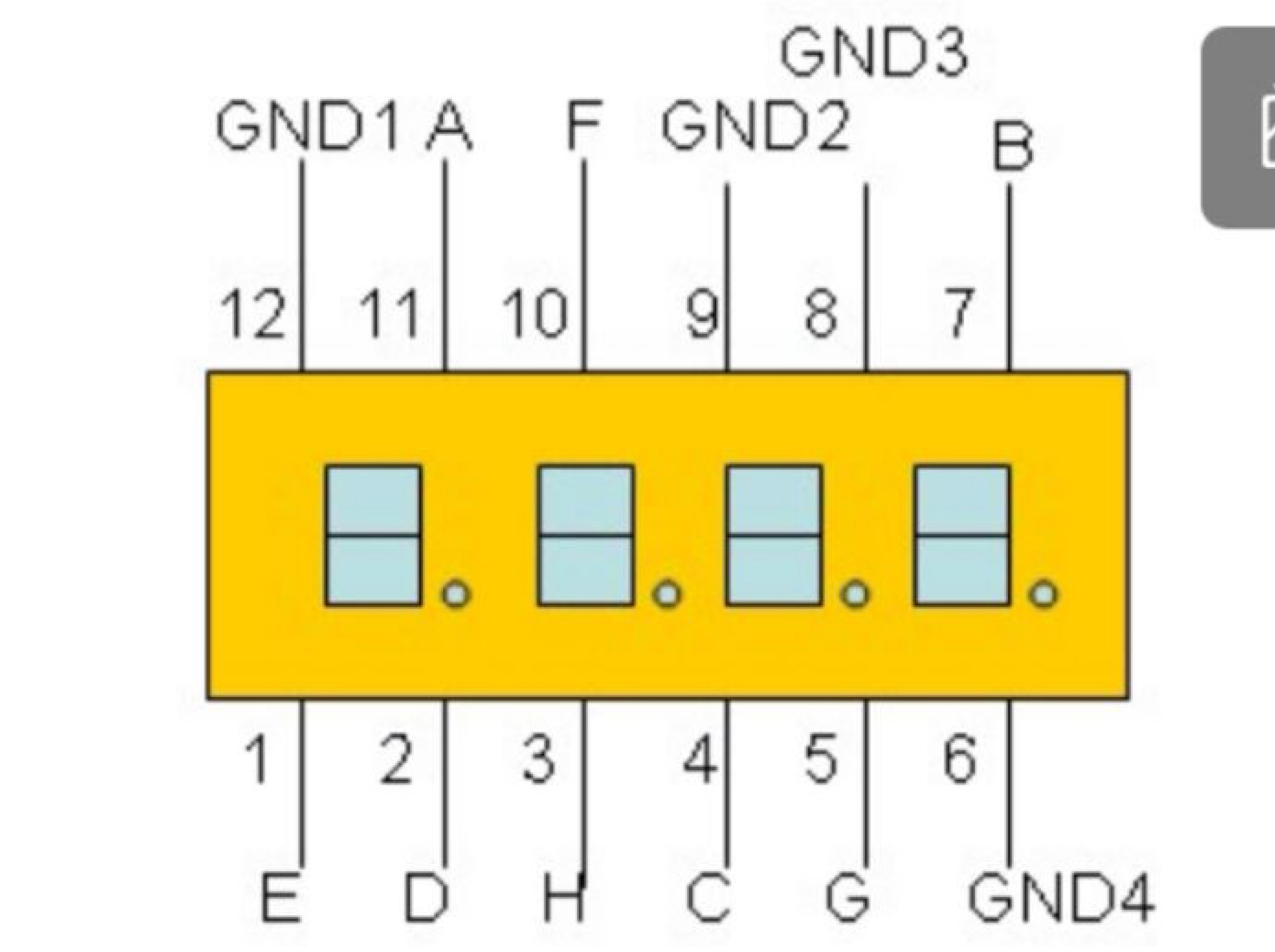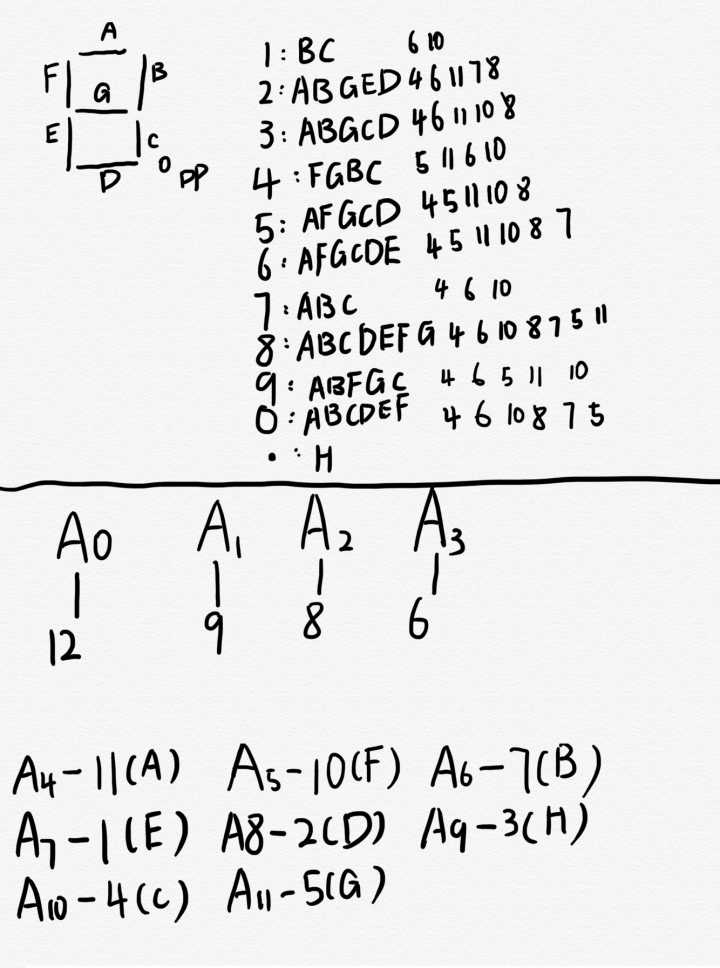# 基于HAL库 STM32F103C8 控制3641ah八位数码管

stm32初学者，在一次比赛中运用stm32控制3641ah八位数码管的数字显示，在这里记录一下学习进度以及学习过程，以便日后回顾。void display(int m)
{ switch(m)
{case 0:
HAL_GPIO_WritePin(GPIOA,GPIO_PIN_4,GPIO_PIN_SET);
HAL_GPIO_WritePin(GPIOA,GPIO_PIN_6,GPIO_PIN_SET);
HAL_GPIO_WritePin(GPIOA,GPIO_PIN_10,GPIO_PIN_SET);
HAL_GPIO_WritePin(GPIOA,GPIO_PIN_8,GPIO_PIN_SET);
HAL_GPIO_WritePin(GPIOA,GPIO_PIN_7,GPIO_PIN_SET);
HAL_GPIO_WritePin(GPIOA,GPIO_PIN_5,GPIO_PIN_SET);
HAL_GPIO_WritePin(GPIOA,GPIO_PIN_11,GPIO_PIN_RESET);
HAL_GPIO_WritePin(GPIOA,GPIO_PIN_9,GPIO_PIN_RESET);

break;
case 1:
HAL_GPIO_WritePin(GPIOA,GPIO_PIN_6,GPIO_PIN_SET);
HAL_GPIO_WritePin(GPIOA,GPIO_PIN_10,GPIO_PIN_SET);
HAL_GPIO_WritePin(GPIOA,GPIO_PIN_4,GPIO_PIN_RESET);
HAL_GPIO_WritePin(GPIOA,GPIO_PIN_5,GPIO_PIN_RESET);
HAL_GPIO_WritePin(GPIOA,GPIO_PIN_7,GPIO_PIN_RESET);
HAL_GPIO_WritePin(GPIOA,GPIO_PIN_8,GPIO_PIN_RESET);
HAL_GPIO_WritePin(GPIOA,GPIO_PIN_9,GPIO_PIN_RESET);
HAL_GPIO_WritePin(GPIOA,GPIO_PIN_11,GPIO_PIN_RESET);
break;
...
}


void displaynumber(double n)
{  double s=n;
if(s>=10000)
{displaymore(n);}
if(s>=1000&&s<10000)
{displaythousand(n);}
if(s>=100&&s<1000)
{displayhundred(n);}
if(s>=10&&s<100)
{displayten(n);}
if(s>=0&&s<10)
{displayone(n);}
if(s<0)
{displayless(n);}
}


void displayone(double m)
{int a,b,c,d,n;
n=m*1000;
a=n/1000;
b=(n-a*1000)/100;
c=(n-a*1000-b*100)/10;
d=(n-a*1000-b*100-c*10);
while(1)
{HAL_GPIO_WritePin(GPIOA,GPIO_PIN_3,GPIO_PIN_RESET);
display(d);
HAL_Delay(t);
HAL_GPIO_WritePin(GPIOA,GPIO_PIN_3,GPIO_PIN_SET);
HAL_GPIO_WritePin(GPIOA,GPIO_PIN_2,GPIO_PIN_RESET);
display(c);
HAL_Delay(t);
HAL_GPIO_WritePin(GPIOA,GPIO_PIN_2,GPIO_PIN_SET);
HAL_GPIO_WritePin(GPIOA,GPIO_PIN_1,GPIO_PIN_RESET);
display(b);
HAL_Delay(t);
HAL_GPIO_WritePin(GPIOA,GPIO_PIN_1,GPIO_PIN_SET);
HAL_GPIO_WritePin(GPIOA,GPIO_PIN_0,GPIO_PIN_RESET);
display(a);
display2();
HAL_Delay(t);
HAL_GPIO_WritePin(GPIOA,GPIO_PIN_0,GPIO_PIN_SET);
}
}


void display2(void)
{HAL_GPIO_WritePin(GPIOA,GPIO_PIN_9,GPIO_PIN_SET);
}